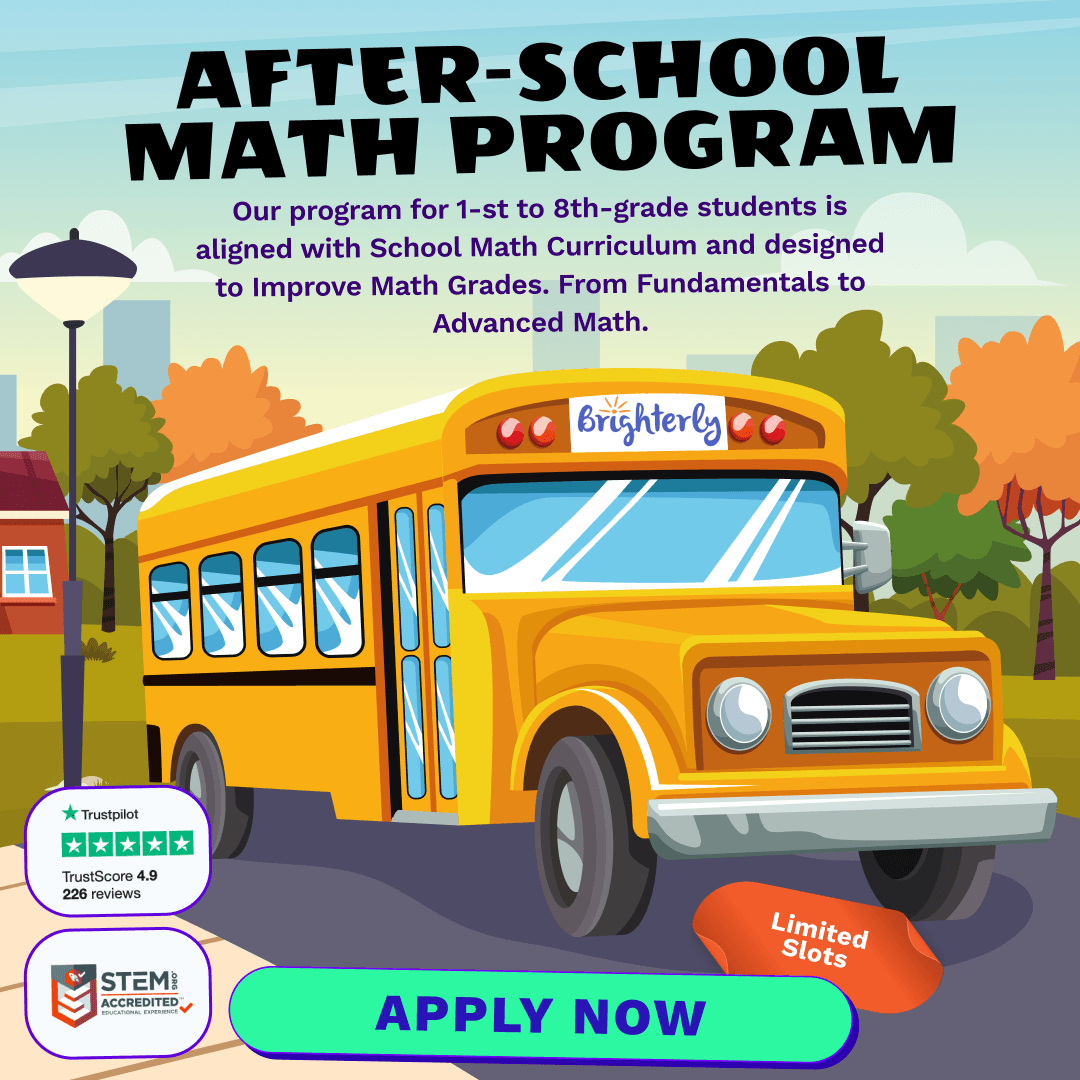# Basic Numbers Math Practice Test for 2nd Grade – [Hard]

Mathematics is an exciting journey, and it begins with understanding the basic numbers, especially for our 2nd graders. At Brighterly, we believe in making math fun, engaging, and understandable for every child. Today, we’re diving deep into the world of 2nd-grade numbers. So buckle up, little mathematicians; we’re about to explore!

## Place Value Magic

Understanding the place value system is the backbone of second-grade math. Imagine numbers as building blocks where each block has a special place.

• Units: This is the rightmost place in a number and represents single units.
• Tens: Just to the left of units, it represents groups of 10.
• Hundreds: And yes, you guessed it! This is a collection of 100 units.

By grasping these basic placements, you can comprehend numbers up to 999. For instance, in the number 256, 2 is in the hundreds place, 5 in the tens, and 6 in the units place.

At 2nd grade, children expand their skills in addition and subtraction, working with two-digit numbers and sometimes even three. Let’s consider a few strategies:

• Regrouping: Also known as carrying and borrowing. When adding or subtracting, you sometimes need to move a number from one place value to another.
• Vertical Stacking: Lining up numbers by their place values makes adding or subtracting a breeze.

## Exploring Word Problems

Word problems are little stories, but instead of a climax, you find a math problem! They’re an introduction to real-world applications of math. Reading comprehension intertwines with mathematical operations, making it a multifaceted learning experience.

Remember, every child’s journey in math is unique. Some might grasp concepts instantly, while others may take a while. The key is patience, practice, and persistence. With Brighterly by your side, every child can shine bright in the world of numbers!

Basic Numbers Practice Test for 2nd Grade

Get ready for math lessons with Brighterly! Crafted meticulously by the expert educators at Brighterly, this test is designed for our young mathematicians who are ready to test their mettle.

1 / 15

What number comes next in the pattern? 100, 200, 400, 800, ...

2 / 15

How many tens are there in the number 758?

3 / 15

Which number is the largest when written in standard form?

4 / 15

Subtract the smallest two-digit number from the smallest three-digit number. What do you get?

5 / 15

Which of the following represents the number 365 in expanded form?

6 / 15

Which of the following is NOT a prime number?

7 / 15

If you add the largest two-digit number to itself, what's the result?

8 / 15

Which number is exactly halfway between 50 and 100?

9 / 15

What's the missing number? 543 - ___ = 200

10 / 15

Which number does NOT belong in the sequence? 3, 6, 9, 12, 15, 18, 22

11 / 15

Which of these numbers is an odd composite number?

12 / 15

If there are 25 dozens of candies in a box, how many individual candies are there?

13 / 15

Which of the following numbers is the smallest?

14 / 15

What number do you get when you multiply the largest one-digit number with itself?

15 / 15

If you arrange the digits 5, 4, and 3 to form the smallest possible number, which number will you get?

0%
Poor Level
Weak math proficiency can lead to academic struggles, limited college, and career options, and diminished self-confidence.
Mediocre Level
Weak math proficiency can lead to academic struggles, limited college, and career options, and diminished self-confidence.
Needs Improvement
Start practicing math regularly to avoid your child`s math scores dropping to C or even D.
High Potential
It's important to continue building math proficiency to make sure your child outperforms peers at school.

After-School Math Programs• Our program for 1st to 8th grade students is aligned with School Math Curriculum.

After-School Math Programs
Our program for 1st to 8th grade students is aligned with School Math Curriculum.